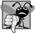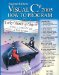# Argument Promotion and Casting

Another important feature of method calls is argument promotionimplicitly converting an argument's value to the type that the method expects to receive in its corresponding parameter. For example, an application can call Math method Sqrt with an integer argument even though the method expects to receive a double argument (but, as we will soon see, not vice versa). The statement

```Console.WriteLine( Math.Sqrt( 4 ) );
```

correctly evaluates Math.Sqrt( 4 ) and prints the value 2.0. The method declaration's parameter list causes C# to convert the int value 4 to the double value 4.0 before passing the value to Sqrt. Attempting these conversions may lead to compilation errors if C#'s promotion rules are not satisfied. The promotion rules specify which conversions are allowedthat is, which conversions can be performed without losing data. In the Sqrt example above, an int is converted to a double without changing its value. However, converting a double to an int truncates the fractional part of the double valuethus, part of the value is lost. Also, double variables can hold values much larger (and much smaller) than int variables, so assigning a double to an int can cause a loss of information when the double value doesn't fit in the int. Converting large integer types to small integer types (e.g., long to int) can also result in changed values.

The promotion rules apply to expressions containing values of two or more simple types and to simple-type values passed as arguments to methods. Each value is promoted to the appropriate type in the expression. (Actually, the expression uses a temporary copy of each valuethe types of the original values remain unchanged.) Figure 7.5 lists the simple types alphabetically and the types to which each can be promoted. Note that values of all simple types can also be implicitly converted to type object. We demonstrate such implicit conversions in Chapter 25, Data Structures.

Figure 7.5. Implicit conversions between simple types.

Type

Conversion types

bool

no possible implicit conversions to other simple types

byte

ushort, short, uint, int, ulong, long, decimal, float or double

char

ushort, int, uint, long, ulong, decimal, float or double

decimal

no possible implicit conversions to other simple types

double

no possible implicit conversions to other simple types

float

double

int

long, decimal, float or double

long

decimal, float or double

sbyte

short, int, long, decimal, float or double

short

int, long, decimal, float or double

uint

ulong, long, decimal, float or double

ulong

decimal, float or double

ushort

uint, int, ulong, long, decimal, float or double

By default, C# does not allow you to implicitly convert values between simple types if the target type cannot represent the value of the original type (e.g., the int value 2000000 cannot be represented as a short, and any floating-point number with digits after its decimal point cannot be represented in an integer type such as long, int or short). Therefore, to prevent a compilation error in cases where information may be lost due to an implicit conversion between simple types, the compiler requires you to use a cast operator (introduced in Section 5.9) to explicitly force the conversion. This enables you to "take control" from the compiler. You essentially say, "I know this conversion might cause loss of information, but for my purposes here, that's fine." Suppose you create a method Square that calculates the square of an integer and thus requires an int argument. To call Square with a double argument named doubleValue, you would write the method call as Square( (int) doubleValue ). This method call explicitly casts (converts) the value of doubleValue to an integer for use in method Square. Thus, if doubleValue's value is 4.5, the method receives the value 4 and returns 16, not 20.25 (which does, unfortunately, result in the loss of information).## Common Programming Error 7 9

Converting a simple-type value to a value of another simple type may change the value if the promotion is not allowed. For example, converting a floating-point value to an integral value may introduce truncation errors (loss of the fractional part) in the result.Visual C# 2005 How to Program (2nd Edition)
ISBN: 0131525239
EAN: 2147483647
Year: 2004
Pages: 600